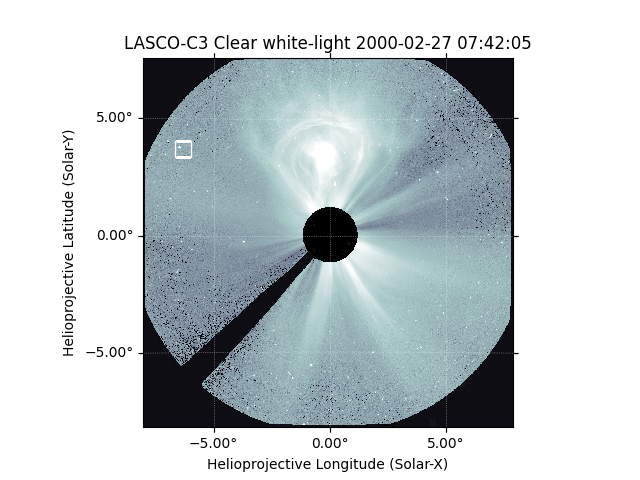# Setting the correct position for SOHO in a LASCO C3 Map¶

How to get the correct location of SOHO using JPL HORIZONS and update the header.

This requires the intallation of the astroquery package and an internet connection. astroquery can be installed ontop of the existing sunpy conda environment: `conda install -c astropy astroquery`

```import matplotlib.pyplot as plt
import numpy as np

import sunpy.map
from sunpy.coordinates.ephemeris import get_body_heliographic_stonyhurst, get_horizons_coord
from sunpy.net import helioviewer

hv = helioviewer.HelioviewerClient()
```

Let’s download a SOHO/LASCO C3 image from Helioviewer which provided pre-processed images and load it into a Map.

```f = hv.download_jp2('2000/02/27 07:42', observatory='SOHO', instrument='LASCO', detector='C3')
lasco = sunpy.map.Map(f)
```

Out:

```Files Downloaded:   0%|          | 0/1 [00:00<?, ?file/s]

2000_02_27__07_42_05_810__SOHO_LASCO_C3_white-light.jp2:   0%|          | 0.00/528k [00:00<?, ?B/s]

2000_02_27__07_42_05_810__SOHO_LASCO_C3_white-light.jp2:   0%|          | 100/528k [00:00<10:01, 878B/s]

2000_02_27__07_42_05_810__SOHO_LASCO_C3_white-light.jp2:  64%|######4   | 340k/528k [00:00<00:00, 1.89MB/s]

```

A user warning let’s you know that there is missing metadata for the observer location. sunpy goes ahead and assumes that the observer is at Earth.

```print(lasco.observer_coordinate)
```

Out:

```<SkyCoord (HeliographicStonyhurst: obstime=2000-02-27T07:42:05.810, rsun=695700.0 km): (lon, lat, radius) in (deg, deg, km)
(0., -7.18539124, 1.48139588e+08)>
```

To check that this worked let’s get the location of Mercury in this exposure and show that it is in the correct location.

```mercury_wrong = get_body_heliographic_stonyhurst(
'mercury', lasco.date, observer=lasco.observer_coordinate)
mercury_hpc_wrong = mercury_wrong.transform_to(lasco.coordinate_frame)
print(mercury_hpc_wrong)
```

Out:

```INFO: Apparent body location accounts for 330.72 seconds of light travel time [sunpy.coordinates.ephemeris]
<Helioprojective Coordinate (obstime=2000-02-27T07:42:05.810, rsun=695700.0 km, observer=<HeliographicStonyhurst Coordinate (obstime=2000-02-27T07:42:05.810, rsun=695700.0 km): (lon, lat, radius) in (deg, deg, m)
(0., -7.18539124, 1.48139588e+11)>): (Tx, Ty, distance) in (arcsec, arcsec, km)
(-23270.61112792, 13370.26466196, 99146796.1462121)>
```

Let’s plot how this looks with the incorrect observer information.

```fig = plt.figure()

# Let's tweak the axis to show in degrees instead of arcsec
lon, lat = ax.coords
lon.set_major_formatter('d.dd')
lat.set_major_formatter('d.dd')
ax.plot_coord(mercury_hpc_wrong, 's', color='white',
fillstyle='none', markersize=12, label='Mercury')
lasco.plot(axes=ax)

plt.show()
```SOHO is actually a halo orbit around the Sun–Earth L1 point, about 1 million km away from the Earth. The following functions queries JPL HORIZONS which includes positions of major spacecraft. This function requires an internet connection to fetch the ephemeris data.

```soho = get_horizons_coord('SOHO', lasco.date)
```

Out:

```INFO: Obtained JPL HORIZONS location for SOHO (spacecraft) (-21) [sunpy.coordinates.ephemeris]
```

For fun, let’s see how far away from the Earth SOHO is by converting to an Earth-centered coordinate system (GCRS).

```print(soho.transform_to('gcrs').distance.to('km'))
```

Out:

```1352065.7329907287 km
```

```lasco.meta['HGLN_OBS'] = soho.lon.to('deg').value
lasco.meta['HGLT_OBS'] = soho.lat.to('deg').value
```

Let’s get the right position now.

```mercury = get_body_heliographic_stonyhurst(
'mercury', lasco.date, observer=lasco.observer_coordinate)
mercury_hpc = mercury.transform_to(lasco.coordinate_frame)
```

Out:

```INFO: Missing metadata for solar radius: assuming the standard radius of the photosphere. [sunpy.map.mapbase]
INFO: Apparent body location accounts for 326.33 seconds of light travel time [sunpy.coordinates.ephemeris]
```

The difference between the incorrect position and the right one is:

```r = np.sqrt((mercury_hpc.Tx - mercury_hpc_wrong.Tx) ** 2 +
(mercury_hpc.Ty - mercury_hpc_wrong.Ty) ** 2)
print(r)
```

Out:

```0.42715952310081085 arcsec
```

Let’s plot the results.

```fig = plt.figure()

# Let's tweak the axis to show in degrees instead of arcsec
lon, lat = ax.coords
lon.set_major_formatter('d.dd')
lat.set_major_formatter('d.dd')
ax.plot_coord(mercury_hpc, 's', color='white', fillstyle='none', markersize=12, label='Mercury')
lasco.plot(axes=ax)

plt.show()
```Total running time of the script: ( 0 minutes 1.781 seconds)

Gallery generated by Sphinx-Gallery